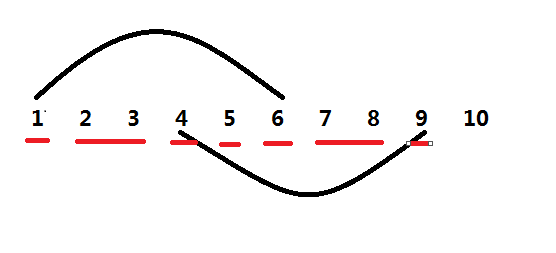IC_QQQ

2019-05-13 10:21:07

### 求逆序对（1,1) ， (2,2) ， (4,1) ， (5,1) ， (6,1) ， (7,2) ， (9,1)

（6,1），（2,2），（9,1），（5,1），（1,1），（7,2），（4,1）

### 代码：

#include<bits/stdc++.h>
#define ll long long
using namespace std;
const int N=1e5+5;
int n,st,tot;
struct aaa{
int x,y;
}Q[N];//记录需要交换的数
int t[2*N],id[2*N];//t:权值。id:代表元
int s[2*N],row[22*N];
ll ans,c[2*N];

int query(int val){//离散化的查询
return lower_bound(row+1,row+1+tot,val)-row;
}

for(;pos<=tot;pos+=pos&-pos) c[pos]+=w;
return;
}

ll sum=0;
for(;pos;pos-=pos&-pos) sum+=c[pos];
return sum;
}

int main(){
scanf("%d",&n);
for(int i=1;i<=n;i++){
scanf("%d%d",&Q[i].x,&Q[i].y);
s[i]=Q[i].x;s[i+n]=Q[i].y;
}
sort(s+1,s+1+2*n);
st=unique(s+1,s+1+2*n)-(s+1);
row[++tot]=s;t[tot]=1;
for(int i=2;i<=st;i++){
if(s[i]-s[i-1]>1){
row[++tot]=s[i-1]+1;
t[tot]=s[i]-s[i-1]-1;
}
row[++tot]=s[i];t[tot]=1;
}
for(int i=1;i<=tot;i++) id[i]=i;
for(int i=1;i<=n;i++){
int x=query(Q[i].x),y=query(Q[i].y);
swap(t[x],t[y]);
swap(id[x],id[y]);
}
for(int i=tot;i>=1;i--){
}